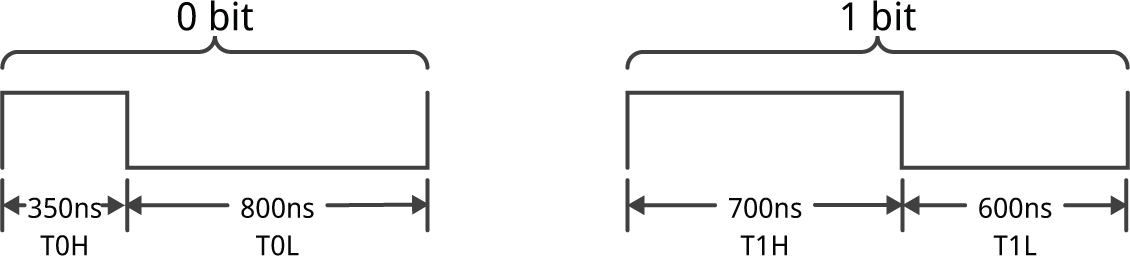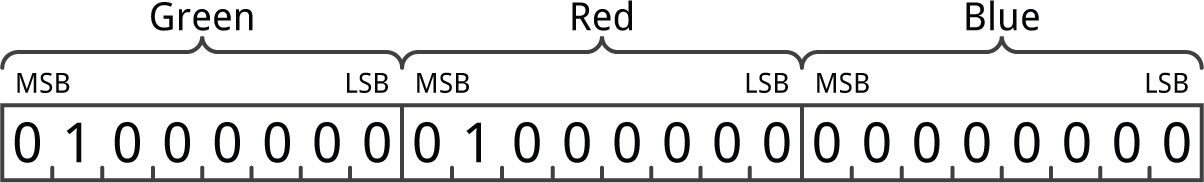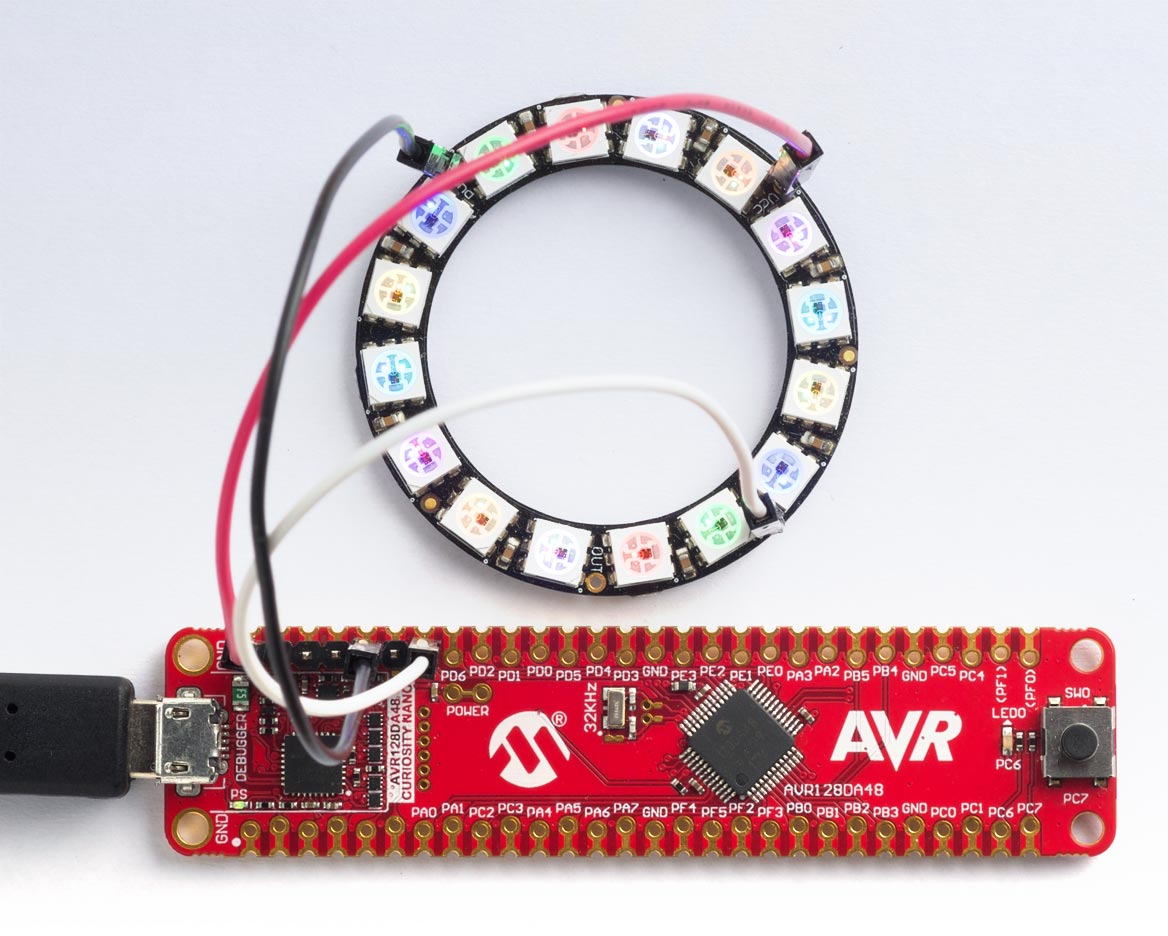## AVR NeoPixel driver using assembler

4th April 2021

NeoPixels use a non-standard protocol consisting of a serial stream of pulses, and the width of each pulse determines whether it is a '0' or a '1'. However the pulses are very short; a zero is defined as having a maximum width of 500ns, which is just 8 cycles on a 16 MHz CPU, or 12 cycles on a 24 MHz CPU. Most NeoPixel libraries therefore use assembler routines tailored to each processor, at least for the low-level pulse generation. This is therefore an excellent application for the built-in assember in uLisp.

### Introduction

NeoPixel is the name given by Adafruit to the WS2812, a chainable RGB LED invented in 2013 by the Shenzhen-based company Worldsemi. You can power them with 5V or 3.3V, and chain them together, tying the data-out pin of one NeoPixel to the data-in of the next one in the chain. There's an excellent overview of NeoPixels on the Adafruit site .

You can get single NeoPixels in a variety of shapes and sizes: Through-hole: 8mm , and 5mm ; SMD: 5050 , 3535 , 2427 , and 1515 . You can also get them already mounted in almost any configuration, including strips, discs, and matrixes.

### The NeoPixel protocol

The datasheet  specifies these timings as the ideal timings for the zero and one bits, and the gaps after each bit:Here is a table showing the number of clock cycles that each of these timings correspond to on processors with different clock frequencies. All the timings have a tolerance of ±150 ns:

 Time 16 MHz 24 MHz T0H 350 ns 5.6 8.4 T0L 800 ns 12.8 19.2 T1H 700 ns 11.2 16.8 T1L 600 ns 9.6 14.4

The most critical time is T0H; at 16 MHz this is just five processor instructions, so it's not even practical to write a NeoPixel driver in C.

The colour for each NeoPixel display is specified by a stream of 24 bits:Here are some implementations for different processors, using the uLisp assemblers.

To use these you call the function neopixel with a list of the 3 colour values for each display, in the order: green, red, blue. Each value can be between 0 and 255, where 255 corresponds to the maximum brightness for that colour.

For example, to set a string of eight NeoPixels to red, green, blue, yellow, cyan, magenta, orange, white:

`(neopixel '(0 64 0  64 0 0  0 0 64  64 64 0  60 0 64  0 64 64  32 64 0  64 64 64))`

I've put spaces in to make the groups of three clearer.

Finally, here's a Lisp program that calls the assembler neopixels routine to create a rotating pattern of white lights of different intensity:

```(defun wave (n)
(let (pix)
(dotimes (x 8) (dotimes (y 3) (push (* 8 x) pix)))
(dotimes (x 8) (dotimes (y 3) (push (- 56 (* 8 x)) pix)))
(loop
(neopixel pix)
(setq pix
(append
(cdddr pix)
(list (first pix) (second pix) (third pix))))
(delay n))))```

The parameter gives the delay between updates of the displays. Try:

`(wave 25)`

#### Delay macro

The key section of code in all these routines is the one to generate a delay of a specified number of clock cycles. To get a delay of n clock cycles you could simply put a series of n \$nop instructions, but that would make the program excessively long. Here's a neater solution:

```  (\$ldi 'r22 4)
loop
(\$dec 'r22)
(\$br 'ne loop)```

This uses r22 as a counter to execute the loop a number of times, specified by the constant n specified in the \$ldi instruction, and the total execution time is n * 3 cycles. To save having to repeat this code four times for the four time intervals we can write it as an assembler macro:

```(defun cycles3 (n)
(list
(\$ldi 'r22 n)
(\$dec 'r22)
(\$br 'ne (- *p* 2))))```

This generates a list of the instruction words which will be inserted in the assembler at the appropriate point.

### ATmega1284P version

The following assembler routine allows you to drive a string of NeoPixel LEDs from one I/O pin on an ATmega1284P board, using uLisp.

Here's the whole neopixel routine, which is based on the structure of the simpler list example Summing the integers in a list. First we define the port and pin we want to use to drive the NeoPixels; in this case PA7:

```(defvar port 0)
(defvar pin 7)```

Here's the cycles3 macro:

```(defun cycles3 (n)
(list
(\$ldi 'r22 n)
(\$dec 'r22)
(\$br 'ne (- *p* 2))))```

And here's the neopixel routine:

```(defcode neopixel (lst)
(\$sbi (+ port 1) pin) ; make pin an output
(\$movw 'x 'r24)
(\$cl 'i)
test
(\$sbiw 'x 0) ; test if x is nil
(\$br 'eq ret)
(\$ld 'zl 'x+)
(\$ld 'zh 'x+)
(\$ldd 'r18 'z 2) ; byte
(\$ldi 'r20 #x80)
nextbit
(\$mov 'r19 'r18)
(\$and 'r19 'r20)
(\$br 'ne one)
zero
(\$sbi (+ port 2) pin)
(cycles3 1)
(\$cbi (+ port 2) pin)
(cycles3 1)
(\$br 'eq next)
one
(\$sbi (+ port 2) pin)
(cycles3 3)
(\$cbi (+ port 2) pin)
(cycles3 1)
next
(\$lsr 'r20)
(\$br 'ne nextbit)
(\$ld 'r18 'x+) ; get the cdr
(\$ld 'r19 'x+)
(\$movw 'x 'r18) ; point to next item
(\$rjmp test)
ret
(\$se 'i)
(\$ret))```

For safety, interrupts are disabled around the routine.

### AVR128DA48 and AVR128DB48 version

The following assembler routine allows you to drive a string of NeoPixel LEDs from one I/O pin on an AVR128DA48 or AVR128DB48 Curiosity Nano board, using uLisp. To test the routine I used an Adafruit NeoPixel ring of 16 RGB NeoPixel LEDs :Here's the whole neopixel routine, which is based on the structure of the simpler list example Summing the integers in a list. First we define the port and pin we want to use to drive the NeoPixels; in this case PD7:

```(defvar port #x0c)
(defvar pin 7)```

Here's the cycles3 macro:

```(defun cycles3 (n)
(list
(\$ldi 'r22 n)
(\$dec 'r22)
(\$br 'ne (- *p* 2))))```

And here's the neopixel routine:

```(defcode neopixel (lst)
(\$sbi port pin) ; make pin an output
(\$movw 'x 'r24)
(\$cl 'i)
test
(\$sbiw 'x 0) ; test if x is nil
(\$br 'eq ret)
(\$ld 'zl 'x+)
(\$ld 'zh 'x+)
(\$ldd 'r18 'z 2) ; byte
(\$ldi 'r20 #x80)
nextbit
(\$mov 'r19 'r18)
(\$and 'r19 'r20)
(\$br 'ne one)
zero
(\$sbi (+ 1 port) pin)
(cycles3 2)
(\$cbi (+ 1 port) pin)
(cycles3 2)
(\$br 'eq next)
one
(\$sbi (+ 1 port) pin)
(cycles3 5)
(\$cbi (+ 1 port) pin)
(cycles3 3)
next
(\$lsr 'r20)
(\$br 'ne nextbit)
(\$ld 'r18 'x+) ; get the cdr
(\$ld 'r19 'x+)
(\$movw 'x 'r18) ; point to next item
(\$rjmp test)
ret
(\$se 'i)
(\$ret))```

For safety, interrupts are disabled around the routine.

1. ^ NeoPixel Überguide on Adafruit.
2. ^ NeoPixel Diffused 8mm Through-Hole LED on Adafruit.
3. ^ NeoPixel Diffused 5mm Through-Hole LED on Adafruit.
4. ^ NeoPixel RGB 5050 LED on Adafruit.
5. ^ Mini 3535 RGB LEDs on Adafruit.
6. ^ NeoPixel Nano 2427 RGB LEDs on Adafruit.